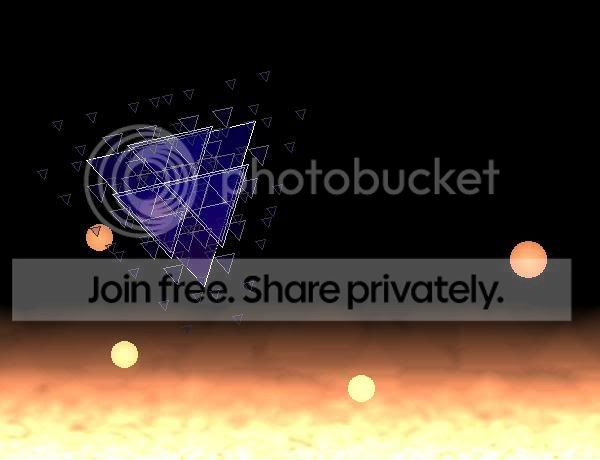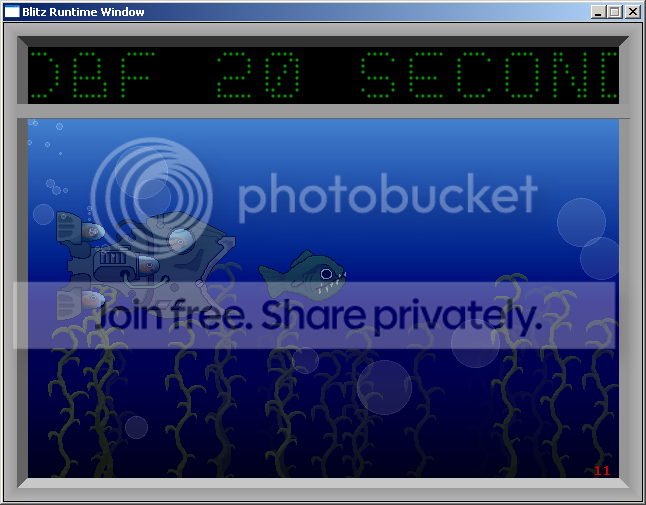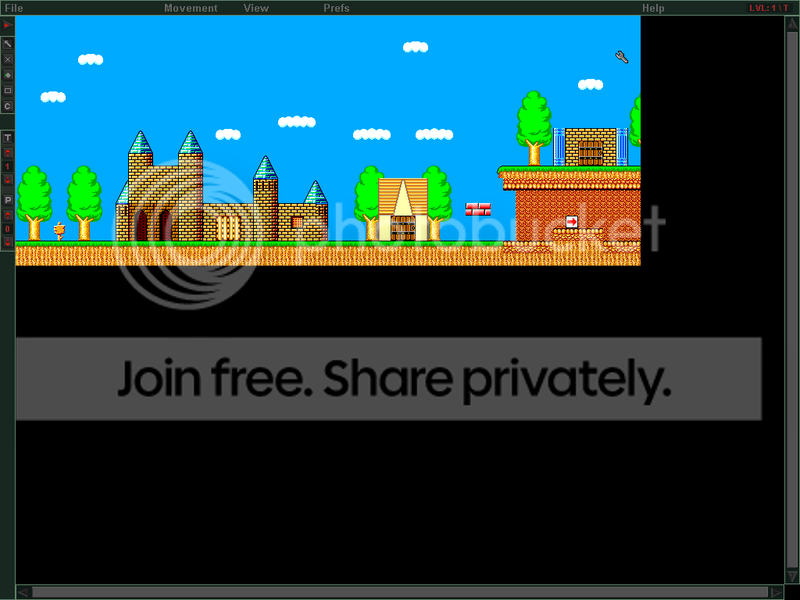-=+=- -=+=- -=+=- -=+=- -=+=- -=+=- -=+=- -=+=- -=+=- -=+=- -=+=- -=+=- -=+=- -=+=- -=+=- -=+=- -=+=- -=+=- -=+=- -=+=- -=+=- -=+=- -=+=- -=+=- -=+=- -=+=- -=+=- -=+=- -=+=- -=+=- (c) WidthPadding Industries 1987 0|698|0 -=+=- -=+=- -=+=- -=+=- -=+=- -=+=- -=+=- -=+=- -=+=- -=+=- -=+=- -=+=- -=+=- -=+=- -=+=- -=+=- -=+=- -=+=- -=+=- -=+=- -=+=- -=+=- -=+=- -=+=- -=+=- -=+=- -=+=- -=+=- -=+=- -=+=-

### mike_g

View Profile, Send Private Message
Recent Posts, Showcases, Articles, Code Snippets, Links, Wed.Workshop, Blog

### Latest Blog Entries

mike_g# RPG Game

12th April 2007

Version 0.01

Heres a very early working version. You can do some stuff like equip items, eat food, use keys to unlock doors, and set off pressure plates.

Key controls for actions are:
L : look
O : open
E : eat
U : use
D : drop
Right clicking *snip*

mike_g# Logic Box

8th November 2006

[img]https://i92.photobucket.com/albums/l15/mikegrundel/Circuit.png[/img]

this is just a screenie of a little puzzle game I started making last night.

Basically you will try to make curcuits that do different things, like the one I made above, using*snip*

### Latest Showcases

mike_g# Lavapit Demo

21st August 2007Heres a demo with bouncing fireballs and stuff. It could do with some sound, but other than that its complete.

mike_g# 20 Second Fish Tank Demo

12th March 2007A short old school demo I made. Can anyone let us know if you get a MAV when you run this? It works fine for me but someone else said that they had a problem with it. Cheers :)

mike_g# Space Balls

7th January 2007A Fast Paced 2D Shooter

mike_g# Tile Up

25th September 20062d Tile editor With lots of features